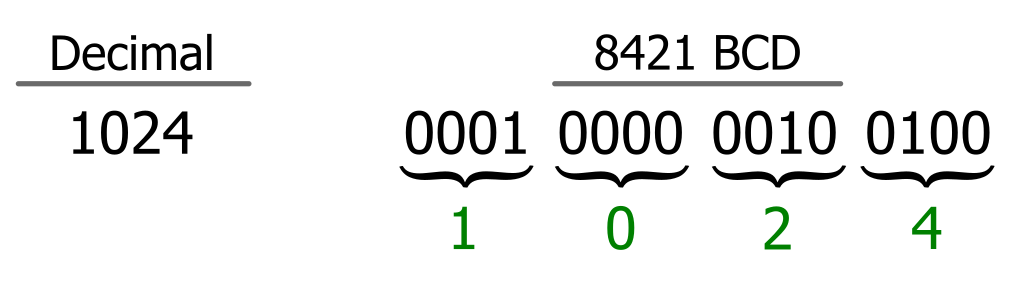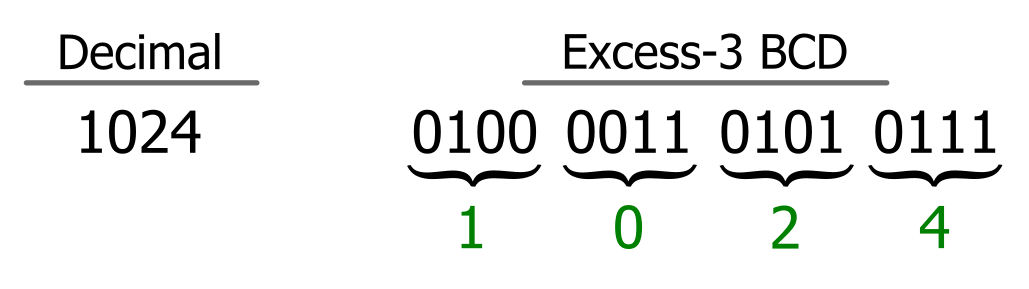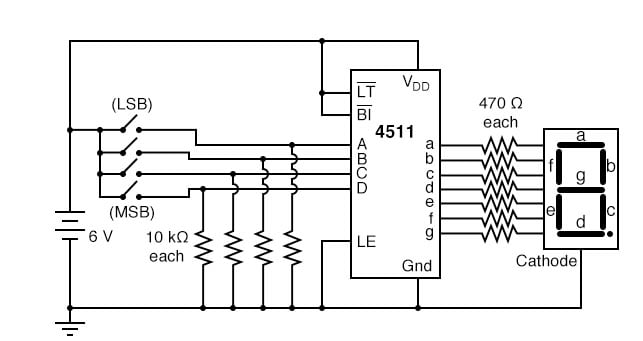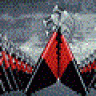Technical Article

# Fundamentals of Binary-Coded Decimal (BCD)

July 07, 2023 by Robert Keim

## This article provides an introduction to the computational number system known as binary-coded decimal or BCD. This numeric encoding scheme is historically significant and still advantageous in certain applications.

Human beings have devised many ways of representing quantities. Electrical engineers regularly work with numbers using the base-10 system (also known as decimal), the base-2 system (binary), and the base-16 system (hexadecimal). The base-2 system has long been preferable for electronic computer systems because its two possible values (one and zero) translate directly into two stable, unambiguous electrical states (on and off).

However, we live in a decimal world; even in the current age of pervasive digital technology, people continue to think and interact using the base-10 system. In the early days of computing technology, scientists sought to alleviate the friction between binary computers and decimal humans by blending these two numerical models into something called binary-coded decimal (BCD).

### What Is Binary-Coded Decimal?

You will often see “binary-coded decimal” written without a hyphen. I insist upon the hyphen, because in my opinion it helps to convey the nature of BCD: binary-coded decimal is primarily a decimal numbering system, and “binary-coded” indicates the form of representation. In other words, the hyphen reminds me that BCD is more decimal than binary.

The defining characteristic of a modern numeral system is the multiplicative relationship between digit positions. In the base-10 system, the “weight” of each digit increases by a factor of ten as you move from right to left. In the base-2 system, the weight increases by a factor of two. In the BCD system, the weight of each digit also increases by a factor of ten—except that a “digit” is now a four-bit binary sequence instead of the zero-through-nine digits that we’re accustomed to. In other words, we’re using a binary sequence to represent decimal digits.

Let’s look at an example in Table 1.

##### Table 1. Binary-coded decimal example
Decimal Binary BCD
132 10000100 0001 0011 0010

The BCD quantity is obtained from the decimal number as follows:

1×102 + 3×101 + 2×100 = 132

The corresponding calculation for the binary number is:

1×27 + 0×26 + 0×25 + 0×24 + 0×23 + 1×22 + 0×21 + 0×20 = 132

To extract the quantity from the BCD number, we do this:

(LLLH)×102 + (LLHH)×101 + (LLHL)×100.

Note that the base of the exponent is ten, as in the decimal system. I used L and H (meaning low and high) instead of ones and zeros to clarify that the expressions in parentheses are not base-10 numbers.

I used L and H also to help convey that the sequences used in BCD are arbitrary. In the example above, I chose the standard binary representations for 1, 3, and 2.

This is a sensible choice, but I could also create a different coding scheme in which the digits 0 through 9 correspond to customized sequences of ones and zeros. Thus, the four-bit binary “digits” in BCD are not necessarily standard base-2 numbers. They are more like symbols—i.e., binary-coded representations of the decimal digits 0 through 9—and I see this as another reason to conceptualize BCD as a primarily decimal numbering system.

### The Many Types of BCD

I just indicated that it is possible to use custom binary sequences for BCD digits, and in fact, many varieties of BCD do not use the standard base-2 equivalents for the digits 0 through 9.

#### 8421 Encoding of BCD Numbers

The BCD number in the example of Table 1 uses 8421 encoding, also called natural BCD, simple BCD, or vanilla BCD. (Okay, I made up the vanilla one.) The name “8421” refers to the quantities assigned to the first four positions in a binary number: 23 = 8, 22 = 4, 21 = 2, 20 = 1. With 8421 encoding, a base-10 number is written using four-bit symbols representing decimal digits, as demonstrated in Table 2.

##### Table 2. Binary-coded decimal 8421 encoding
 Decimal Digits BCD 8 4 2 1 0 0 0 0 0 1 0 0 0 1 2 0 0 1 0 3 0 0 1 1 4 0 1 0 0 5 0 1 0 1 6 0 0 1 1 7 1 0 0 0 8 1 0 0 1

Using that method, Figure 1 demonstrates how to represent the decimal value of 1024 using the 8421 encoding.##### Figure 1. Representing decimal 1024 using the 8421 encoding method for BCD numbers

In other encoding schemes, the decimal-to-binary correspondence doesn’t follow the natural mathematical relationship between base-10 and base-2 that is found in the 8421 encoding scheme.

#### Excess-3 Encoding of BCD Numbers

For example, Figure 2 demonstrates the decimal value of 1024 represented using excess-3 encoding:##### Figure 2. Representing decimal 1024 using the Excess-3 encoding method for BCD numbers

Excess-3 encoding facilitates arithmetic operations and is still relevant to recent research. A 2016 paper, for instance, reports on an electro-optical method of converting Excess-3 to 8421 and describes Excess-3 as “one of the most important codes used for efficient data storage and transmission.”

#### Other BCD Encoding Methods

Quite a few other four-bit encoding schemes exist, and it’s worth noting that there is no law stating that BCD digits must use only four bits. In “packed BCD,” two four-bit BCD digits are packed into one byte. In “unpacked BCD,” however, one byte holds only one digit, such that you could use more than four bits per digit without consuming additional memory.

### BCD Applications

In computing environments, binary-coded decimal is not as prominent as it used to be. Nowadays, it seems to appear most frequently in the context of BCD-to-seven-segment decoders, as illustrated in the circuit of Figure 3.##### Figure 3. The 4511 IC is a BCD-to-seven-segment decoder. Image used courtesy of Lessons In Electronic Circuits by Tony R. Kuphaldt

These devices interpret four input logic signals (A, B, C, and D) as a BCD digit and generate the drive signals needed to produce that decimal digit on a seven-segment LCD or LED display. This application highlights one of BCD’s advantages: it handles numerical data in a way that simplifies conversion to human-readable forms.

However, BCD has another advantage that gives it enduring importance beyond the realm of low-cost display technology: it provides direct equivalents to base-10 numbers with fractional parts (i.e., with digits to the right of the decimal point), and it, therefore, avoids the loss of precision that can occur when converting between number systems with different bases.

Binary-coded decimal requires more memory and typically more processing resources compared to normal binary. However, in some applications, BCD’s enhanced precision outweighs its circuitry costs.

I assume that the cost/benefit picture improved as microelectronic components continued to decrease in size and price, and indeed, by 2007, researchers could state that BCD arithmetic was “preferred” for processing decimal data in scientific, commercial, and financial applications.

### Continuing Research in Binary-coded Decimal

I like the mathematically intuitive nature of binary-coded decimal, and I’m glad to see that it is still an active subject of technical research. The links below, all of which have abstracts if you can’t access the full article, will give you an idea of BCD’s evolving role in modern electrical engineering.

1 Comment•daba1955 July 10, 2023

For years, a major PLC manufacturer had an instruction to read a 4-bit binary input, and decode it into decimal, throwing away “bad” numbers generated by non-decimal interpretations of the bit patterns. I think the instruction generated a minor fault (which could be interrogated) if such a situation existed.

Then they introduced a newer PLC range, which had the same instruction, but interpreted “1010” as 10, “1011” as 11, etc. up to “1111” as 15, with no “errors” being reported.

So, not a Binary to Decimal conversion, but a Hex to Decimal Conversion.

It really makes me mad that over 30 years of great service is thrown under the bus….

Like.